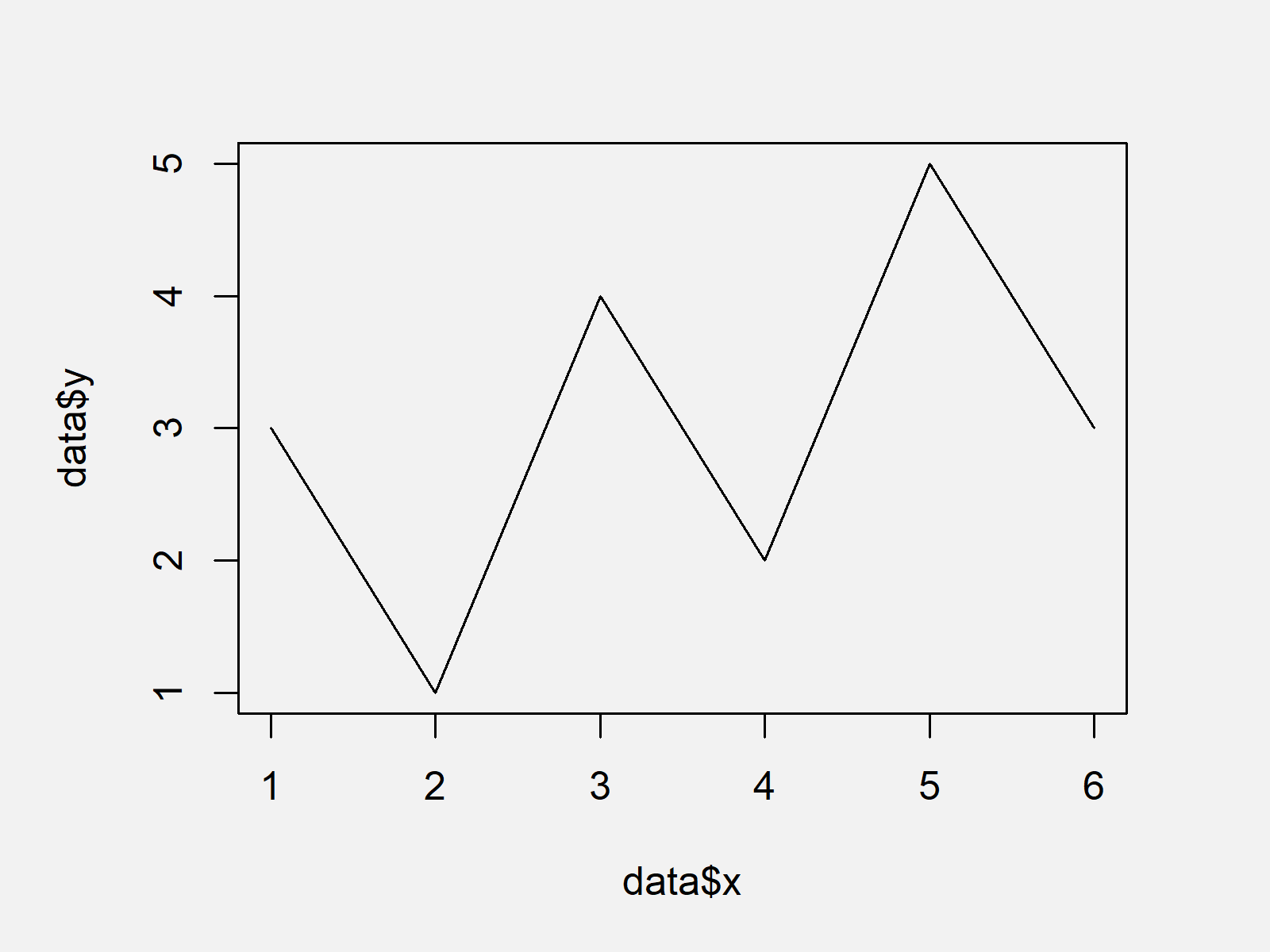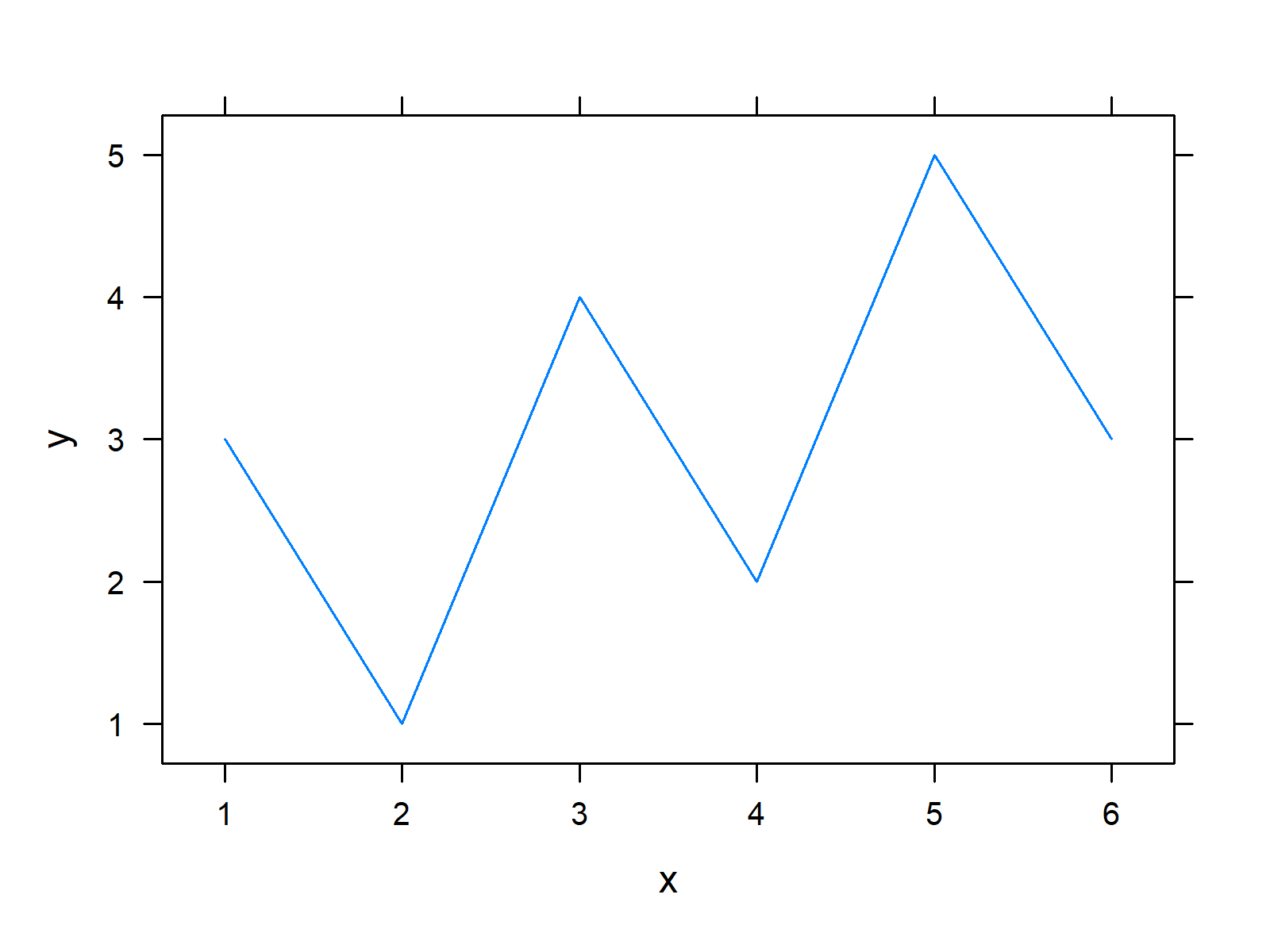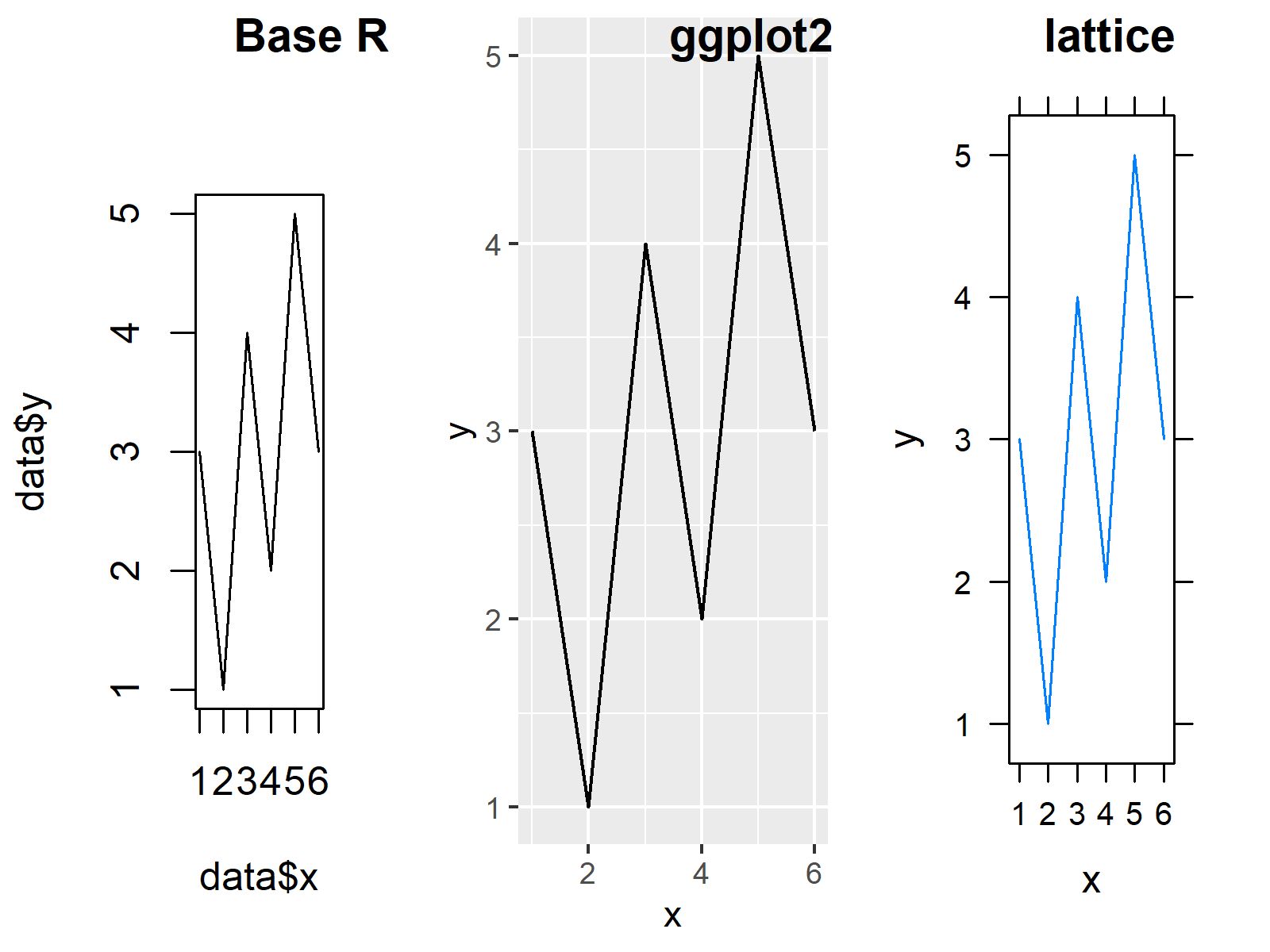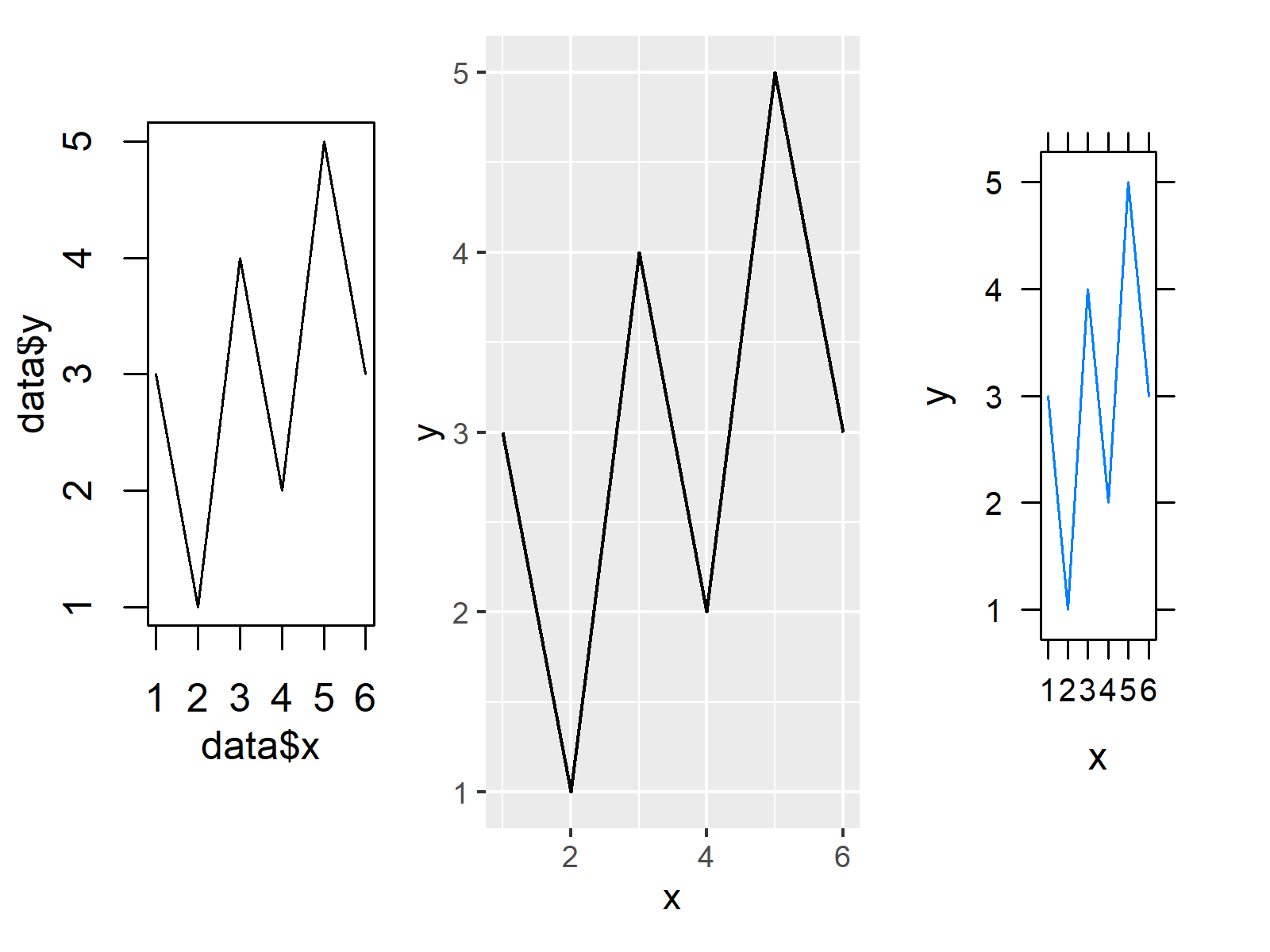# Combine Base R, ggplot2 & lattice Plots (2 Examples)

This article shows how to create a grid of Base R, ggplot2, and lattice plots in the R programming language.

The article will contain two examples for the creation of a grid of Base R, ggplot2, and lattice plots. To be more precise, the post looks as follows:

## Exemplifying Data, Software Packages & Basic Plot

We’ll use the data below as basement for this tutorial:

```data <- data.frame(x = 1:6,                           # Create example data
y = c(3, 1, 4, 2, 5, 3))
data                                                  # Print example data```As you can see based on Table 1, our exemplifying data is a data frame containing six observations and the two columns “x” and “y”. The variable x is an integer and the variable y has the numeric class.

Now, we can plot the data using Base R as shown below:

`plot(data\$x, data\$y, type = "l")                      # Create Base R plot`In Figure 1 it is shown that we have created a line plot using the basic installation of the R programming language by running the previous R programming code.

Next, we have to save this plot in a data object using the recordPlot function:

`my_base <- recordPlot()                               # Save Base R plot`

Next, we have to create a ggplot2 plot. In order to use the functions of the ggplot2 package, we also need to install and load ggplot2 to RStudio:

```install.packages("ggplot2")                           # Install & load ggplot2 package
library("ggplot2")```

We can now create a ggplot2 plot object as shown below:

```my_ggplot <- ggplot(data, aes(x, y)) +                # Create ggplot2 plot
geom_line()
my_ggplot                                             # Save ggplot2 plot```The output of the previous code is shown in Figure 2 – A ggplot2 line graph.

To be able to create a lattice plot, we’ll also have to install and load the lattice package to R:

```install.packages("lattice")                           # Install & load lattice
library("lattice")```

Next, we can create a lattice line plot using the xyplot function as shown in the following R syntax:

```my_lattice <- xyplot(y ~ x, data = data, type = "l")  # Create lattice plot
my_lattice                                            # Save lattice plot```The lattice plot that was drawn by the previous R code is shown in Figure 3.

In the following examples, I’ll explain how to combine our three graphs in a single grid of plots. Keep on reading!

## Example 1: Create Grid of Plots Using cowplot Package

In Example 1, I’ll explain how to use the cowplot package to draw a grid of plots containing Base R, ggplot2, and lattice graphics.

If we want to use the functions of the cowplot package, we also have to install and load cowplot:

```install.packages("cowplot")                           # Install cowplot package

Finally, we can use the plot_grid function to draw our plots side-by-side. Note that we are using the hjust and labels arguments to add a title to each plot in our grid:

```plot_grid(my_base,                                    # Create grid of plots
my_ggplot,
my_lattice,
ncol = 3,
hjust = - 1.5,
labels = c("Base R", "ggplot2", "lattice"))```Figure 4 shows the output of the previous R code: A grid of Base R, ggplot2, and lattice graphics.

## Example 2: Create Grid of Plots Using ggplotify & patchwork Packages

In Example 2, I’ll demonstrate how to use the ggplotify and patchwork packages to create a plot composition containing Base R, ggplot2, and lattice plots.

First, we need to install and load the ggplotify package to RStudio:

```install.packages("ggplotify")                         # Install ggplotify package

We then have to install and load the patchwork add-on package, if we want to use the corresponding functions:

```install.packages("patchwork")                         # Install & load patchwork
library("patchwork")```

Now, we can use the as.ggplot function of the ggplotify function in combination with the typical patchwork syntax to draw a grid of plots:

```as.ggplot(~plot(data\$x, data\$y, type = "l")) +        # Create grid of plots
my_ggplot +
as.ggplot(my_lattice)```As you can see in Figure 5, the previous R code has created a composition of our three plots.

## Video, Further Resources & Summary

Have a look at the following video on my YouTube channel. In the video, I’m explaining the content of this tutorial in RStudio:

Please accept YouTube cookies to play this video. By accepting you will be accessing content from YouTube, a service provided by an external third party.If you accept this notice, your choice will be saved and the page will refresh.

In addition, you may read some of the related tutorials on my website. I have published numerous articles about related topics such as graphics in R and ggplot2.

In this tutorial you have learned how to draw a grid of Base R, ggplot2, and lattice plots in the R programming language. Let me know in the comments below, in case you have further questions.

Subscribe to the Statistics Globe Newsletter